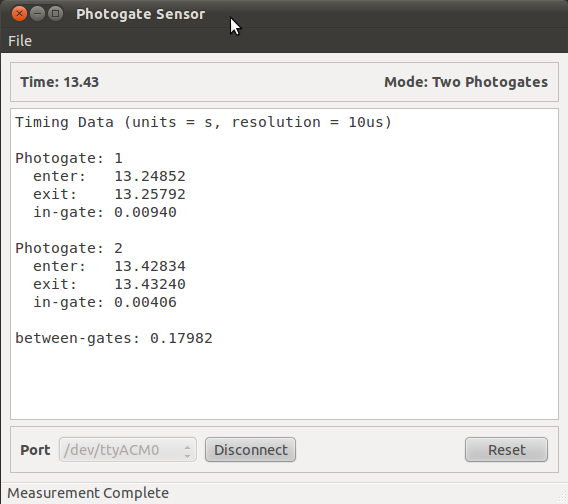# Freefall - calculating g¶

Equation for calculating acceleration due to gravity is:

$g = \frac{v \mathrm{2} - v \mathrm{1}}{\mathrm{\Delta t}}$

where $$g$$ is the acceleration due to gravity, $$v1$$ is the velocity in gate 1, $$v2$$ is the velocity in gate 2 and $$\Delta t$$ is the time between gates.

## 1) Setting up the photogates¶

On a single support stand , place the two photogates a distance apart. Make sure that the top (first) photogate is connected to the Photogate 1 position on the Arduino Shield.## 2) Software¶

Set-up the software as described in the previous section Using the photogate software.

## 3) Sample data¶

In the example shown below, we used a 0.5” wide metal block (12.7mm). Hold the object slightly above the first photogate and release to drop it between the two gates. Save data for analysis.## 4) Data analysis¶

• From time in gates, calculate velocity, $$v$$, of falling object:

• $$v$$ = object length (m) / time in gate (s)
• $$v1$$ = 0.0127/0.00940 = 1.351 $$\mathrm{m}/\mathrm{s}$$
• $$v2$$ = 0.0127/0.00406 = 3.128 $$\mathrm{m}/\mathrm{s}$$
• From equation, g is given by:

$g \approx \frac{3.128-1.351}{0.17982} \approx 9.88 \mathrm{m}/\mathrm{s}$

Footnotes

  Additional equipment from Carolina Biologicals. Support Stand $15.50, Part # 707146 and Clamp Holder,$9.90, Part # 707310